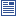Accessibility options:

# Matrix arithmetic resources

Show me all resources applicable to

### Community Project (1)Mathematical Tools for Physical Sciences and Systems Biology (SOURCE)
A zip file containing the LaTex source files and metatdata for the leaflet - a summary of common mathematical definitions and properties used in the Physical Sciences and Systems Biology contributed to the mathcentre Community Project by Dr Morgiane Richard, University of Aberdeen and reviewed by Mamen Romano and Ian Stansfield, University of Aberdeen.

### Facts & Formulae Leaflets (1)Mathematical Tools for Physical Sciences and Systems Biology
This leaflet is a summary of common mathematical definitions and properties used in the Physical Sciences and Systems Biology contributed to the mathcentre Community Project by Dr Morgiane Richard, University of Aberdeen and reviewed by Mamen Romano and Ian Stansfield, University of Aberdeen.

### Quick Reference (8)Addition, subtraction and scalar multiplication of matrices
Matrices 3: This leaflet explains the conditions under which it is possible to add or subtract two matrices. It explains how to perform addition and subtraction when this is possible. It also explains how a matrix can be multiplied by a number. There is an accompanying video tutorial.Matrices - what is a matrix ?
Matrices 1: This leaflet explains what is meant by a matrix, explains the notation used to describe matrices, and introduces some special types of matrix. There is an accompanying video tutorial.Multiplying matrices
This leaflet explains how to multiply two matrices together. (Engineering Maths First Aid Kit 5.3)Multiplying matrices 1
Matrices 5: This leaflet introduces the conditions under which matrices can be multiplied. It shows how matrix multiplication is carried out whenever this is possible. There is an accompanying video tutorial.Multiplying matrices 2
Matrices 6: This leaflet continues to explore the conditions under which matrices can be multiplied. It shows how matrix multiplication is carried out whenever this is possible. There is an accompanying video tutorial.Symmetric matrices and the transpose of a matrix
Matrices 2: This leaflet explains what is meant by a symmetric matrix and the transpose of a matrix. There is an accompanying video tutorial.The inverse of a 2x2 matrix
This leaflet explains what is meant by the inverse of a 2x2 matrix and how this can be found using a formula. (Engineering Maths First Aid Kit 5.4)The inverse of a matrix
This leaflet explains what is meant by the inverse of a matrix and how this can be calculated. (Engineering Maths First Aid Kit 5.5)

### Test Yourself (3)Maths EG
Computer-aided assessment of maths, stats and numeracy from GCSE to undergraduate level 2. These resources have been made available under a Creative Common licence by Martin Greenhow and Abdulrahman Kamavi, Brunel University.Matrices Test 01 (DEWIS)
Five questions on matrix arithmetic testing matrix addition, scalar multiplication, transpose of a matrix and matrix multiplication. DEWIS resources have been made available under a Creative Commons licence by Rhys Gwynllyw & Karen Henderson, University of the West of England, Bristol.Matrix arithmetic - Numbas
Three questions on linear combinations and products of matrices. Numbas resources have been made available under a Creative Commons licence by Bill Foster and Christian Perfect, School of Mathematics & Statistics at Newcastle University.

### Video (5)Addition, subtraction and scalar multiplication of matrices
Matrices 3: This video tutorial explains the conditions under which it is possible to add or subtract two matrices. It explains how to perform addition and subtraction when this is possible. It also explains how a matrix can be multiplied by a number. There is an accompanying help leaflet.Matrices - what is a matrix ?
Matrices 1: This video tutorial explains what is meant by a matrix, explains the notation used to describe matrices, and introduces some special types of matrix. There is an accompanying help leaflet.Multiplying matrices 1
Matrices 5: This video tutorial introduces the conditions under which matrices can be multiplied. It shows how matrix multiplication is carried out whenever this is possible. There is an accompanying help leaflet.Multiplying matrices 2
Matrices 6: This video tutorial continues to explore the conditions under which matrices can be multiplied. It shows how matrix multiplication is carried out whenever this is possible. There is an accompanying help leaflet.Symmetric matrices and the transpose of a matrix
Matrices 2: This video tutorial explains what is meant by a symmetric matrix and the transpose of a matrix. There is an accompanying help leaflet.

### Video with captions which require edits (5)Addition, subtraction and scalar multiplication of matrices
Matrices 3: This video tutorial explains the conditions under which it is possible to add or subtract two matrices. It explains how to perform addition and subtraction when this is possible. It also explains how a matrix can be multiplied by a number. There is an accompanying help leaflet.Matrices - what is a matrix ?
Matrices 1: This video tutorial explains what is meant by a matrix, explains the notation used to describe matrices, and introduces some special types of matrix. There is an accompanying help leaflet.Multiplying matrices 1
Matrices 5: This video tutorial introduces the conditions under which matrices can be multiplied. It shows how matrix multiplication is carried out whenever this is possible. There is an accompanying help leaflet.Multiplying matrices 2
Matrices 6: This video tutorial continues to explore the conditions under which matrices can be multiplied. It shows how matrix multiplication is carried out whenever this is possible. There is an accompanying help leaflet.Symmetric matrices and the transpose of a matrix
Matrices 2: This video tutorial explains what is meant by a symmetric matrix and the transpose of a matrix. There is an accompanying help leaflet.

Website design by Pink Mayhem, Leicester